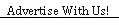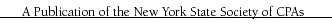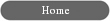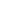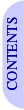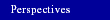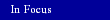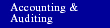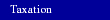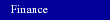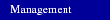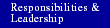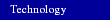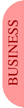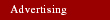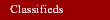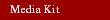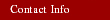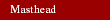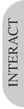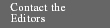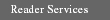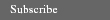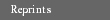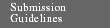Valuing Employee Stock Options: A Binomial Approach Using Microsoft Excel By Joseph A. D’Urso JULY 2005 - There has been substantial controversy ever since FASB first proposed the expensing of employee stock options. Part of the controversy is attributable to disagreement over how options should be valued. Historically, Wall Street has valued options using models such as Black-Scholes. Such models are primarily applicable to short-term publicly traded options, however, and may not be the best approach to valuing employee stock options, which tend to be longer term, nontransferable, and subject to various vesting requirements and forfeiture rules. A recently discussed alternative endorsed by the current FASB proposal is the use of a “lattice” binomial model which takes into consideration those characteristics associated with employee stock options. It can be tailored to a company granting such options. This article will attempt to demonstrate such a model using simple formulas in an Excel spreadsheet, which can be downloaded from www.cpajournal.com/down.htm. The author’s purpose is not to present the most comprehensive approach, but rather to provide food for thought to individuals considering an alternative method for valuing their company’s employee stock options. Example Consider a business, Example Company, with the following characteristics of its employee stock option plan: Market price of stock      \$6.00 Exercise price                  \$6.00 Option term                     5 years Risk-free rate                   4% Volatility                          10% Vesting                            2-year cliff vesting Dividends                         None The first step in the valuation process is to create a standard (i.e., not modified) binomial model. The Cox, Ross, and Rubinstein (CRR) model is easy to construct and is probably the most widely accepted and popular binomial model. For those interested in the theory behind the model, there are numerous books and articles on the subject, including Cox, Ross, and Rubinstein’s original 1979 paper, “Option Pricing: A Simplified Approach,” published in the Journal of Financial Economics. This author’s approach to creating CRR models is similar to that found in Financial Modeling Using Excel and VBA, by Chandan Sengupta (Wiley, 2004). To set up the CRR model in Microsoft Excel, enter the data as shown in Exhibit 1, cells A7 to B12. Then, enter the labels as shown in cells A16 to A21. Finally, enter the following formulas in the corresponding cells: B17 =B11/B12 B18 =(EXP(B10*SQRT(B17))) B19 =1/B18 B20 =(EXP(B9*B17)-B19)/(B18-B19) B21 =EXP(-B9*B17) If the formulas are entered correctly, the data range from B17 to B21 should match that shown in Exhibit 1. The next step is to highlight the range A17 to B21, then select the menu item Insert>Name>Create and click the “left column” box. Follow the same steps with the range A8 to B8. This will assign a name to these cells, making it easier to copy formulas and set up the binomial trees. Moreover, it will allow the formulas to be self-explanatory, as these descriptive names will be used in the formulas instead of the usual cell references. The next step is to set up a “stock price tree.” In accordance with binomial theory, the stock price tree will generate various stock prices (i.e., up and down prices at each step) over the life of the option. (The completed stock price tree is shown in Exhibit 1.) In order to generate the stock price tree, enter the “Step 0–5” labels in cells A28 to G28, and the “Time” label in cell A29. In cell B29, enter the formula “=B28*Time_interval” and copy it across to cell G29. This step is simple in this example, but it becomes important when generating larger trees where the year is divided into many shorter intervals. In cell A31, type the label “Stock Price” and in cell B31 enter “=B7”. In cell C31, enter the formula “=B31*Up_movement” and copy it across to cell G31. In cell C32, enter the formula “=B31*Down_movement” and copy it across to cell G32. Next, copy cell D32 down one row, copy cell E32 down two rows, and so forth, so that a triangular tree is generated. The third step is to construct the “option tree,” following a process known as “backward induction,” which starts at the end of the tree (i.e., at expiration) and works back to the present time. At expiration, a stock option is equal to the greater of its intrinsic value (the market price of the stock at expiration, less the exercise price) or zero; an option can’t have a negative value. To reflect this, enter the formula “=MAX(0,(G31-Exercise_price))” in cell G40, then copy it down to cell G45. According to binomial theory, the amounts in the prior steps represent the discounted expected value of the future upper and lower option values. To reflect this, in cell B40 enter the formula “=(Up_movement_probability*C40+(1-Up_movement_probability)*C41)*Discount_factor” and copy it across to cell F40. Finally, copy cell C40 down one row, copy cell D40 down two rows, and so forth, so that a triangular tree is generated. The end result is a standard binomial model that can be tailored to any company. The model can be validated through a comparison to the Black-Scholes model; the more steps that are utilized, the closer the results should approximate the Black-Scholes model. In this example, it turns out that the Black-Scholes model happens to yield exactly the same amount (\$1.21). Modified Example The final step is to now modify the model to fit a specific company. Continuing with the example above, the two-year cliff vesting assumption and the company’s past exercise history must be considered. Assume that during the last five years of stock option exercises, employees have exercised their options, on average, when the market price exceeded their exercise price by 10%. Thus, it is assumed that the employees will exercise their options as soon as the market price factor (i.e., the current price divided by the exercise price) exceeds 1.10. Employees can only exercise their options, however, once they are vested. These factors can be integrated as follows. First, the exercise factor (1.10) should be input in cell B13. The minimum market price that will trigger the option to be exercised can be calculated by inserting the formula “=B13*Exercise_price”. These cells have been inserted into the spreadsheet as shown in Exhibit 2. Column E represents the first year that employees are vested and free to exercise their options. The formula with “=IF(E31>Minimum_mkt_price, E31-Exercise_price,(Up_movement_probability*F40+(1-Up_movement_probability)*F41)*Discount_factor)” should be inserted in cell E40. This formula tests whether the stock will be exercised, and if it will, the intrinsic value is inserted into the cell. Copy this formula across into cell F40. Then, copy cell E40 down to cell E43 and copy cell F40 down to cell F44. The resulting option tree for the final modified binomial model should match that shown in Exhibit 2. It is interesting to note that although the upper year-one market price from the stock price tree of \$6.63 exceeds the minimum price of \$6.60, the model does not treat the option as exercised because it is not yet vested. Note that after considering the low “exercise factor” and vesting rules, the option price has dropped from \$1.21 to \$0.88: a reduction of 27%. This is the main advantage of using this type of model: Vesting rules and past employee practices can easily be factored in. Although the example above has been kept simple for demonstration purposes, the CRR binomial model can be modified to conform to a specific company’s exercise history and vesting requirements. The formulas can be expanded and edited within the Excel spreadsheet. Joseph A. D’Urso, CPA, CMA, CFM, is director of accounting at a publicly held biotechnology company in Princeton, N.J.The CPA Journal is broadly recognized as an outstanding, technical-refereed publication aimed at public practitioners, management, educators, and other accounting professionals. It is edited by CPAs for CPAs. Our goal is to provide CPAs and other accounting professionals with the information and news to enable them to be successful accountants, managers, and executives in today's practice environments.

©2009 The New York State Society of CPAs. Legal Notices